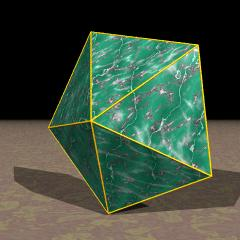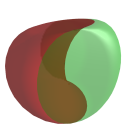Acronym peppy, 5pyr, J2 Name pentagonal pyramid `© ©` Circumradius sqrt[(5+sqrt(5))/8] = 0.951057 Vertex figures , [3,3,5] General of army (is itself convex) Colonel of regiment (is itself locally convex) Dual (topologically selfdual, in different orientation) Dihedral angles between {3} and {3}:   arccos(-sqrt(5)/3) = 138.189685° between {3} and {5}:   arccos(sqrt[(5+2 sqrt(5))/15]) = 37.377368° Confer general pyramids: n/d-py   related Johnson solids: pedpy   gyepip   mibdi   teddi   aud   mabaud   pabaud   taud   uniform relative: ike   general polytopal classes: Johnson solids   segmentohedra   fundamental lace prisms ExternallinksAs abstract polytope peppy is isomorph to stappy, thereby replacing the base pentagon by a pentagram.

This polyhedron is an edge-faceting of the icosahedron (ike).

Incidence matrix according to Dynkin symbol

```ox5oo&#x   → height = sqrt((5-sqrt(5))/10) = 0.525731
(pt || {5})

tip  o. o.    | 1 * | 5 0 | 5 0
base .o .o    | * 5 | 1 2 | 2 1
--------------+-----+-----+----
lace oo5oo&#x | 1 1 | 5 * | 2 0
base .x ..    | 0 2 | * 5 | 1 1
--------------+-----+-----+----
coat ox ..&#x | 1 2 | 2 1 | 5 *
base .x5.o    | 0 5 | 0 5 | * 1
```